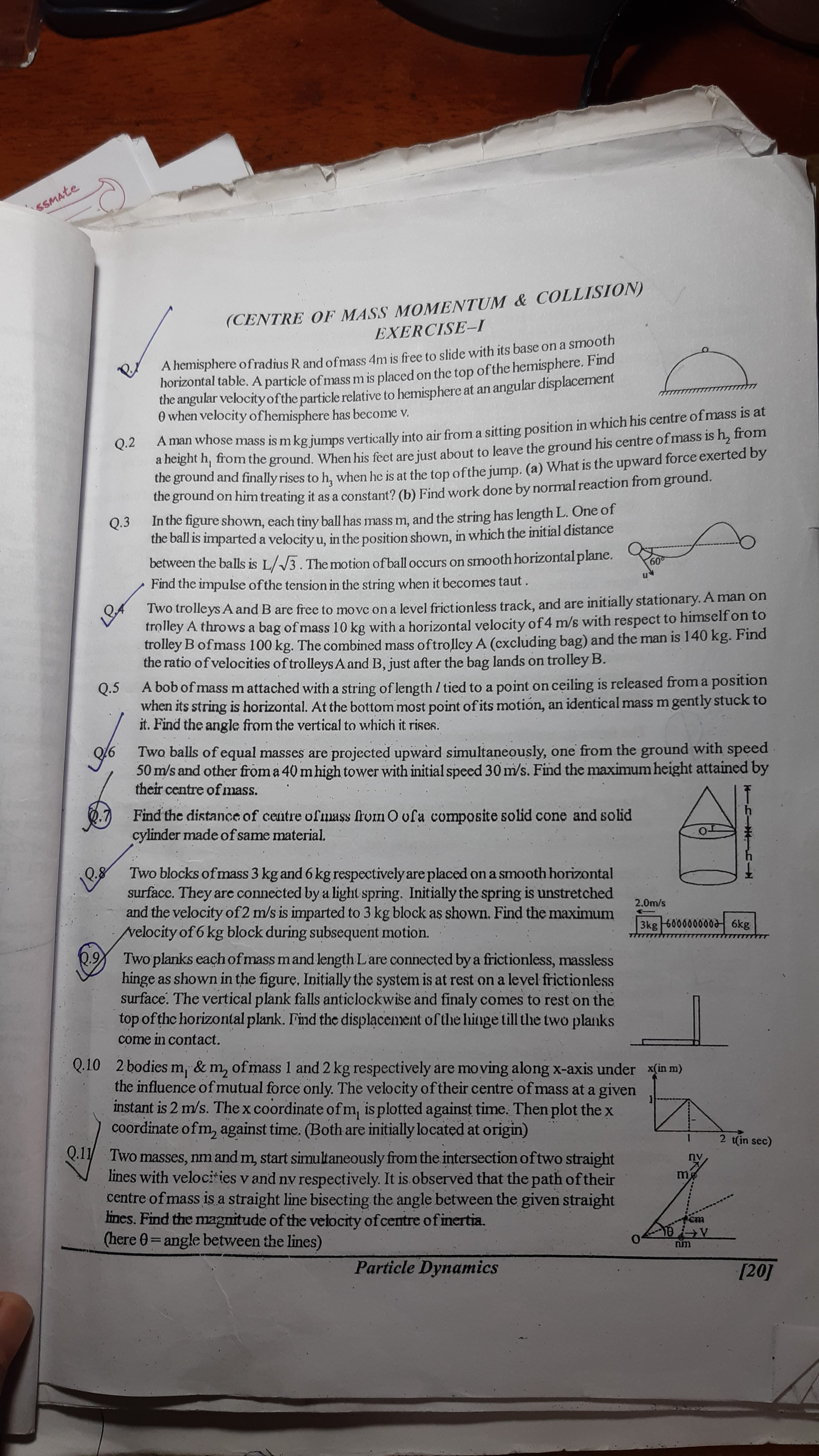# Doubt from conservation of momentum

Question no 1,2 and 14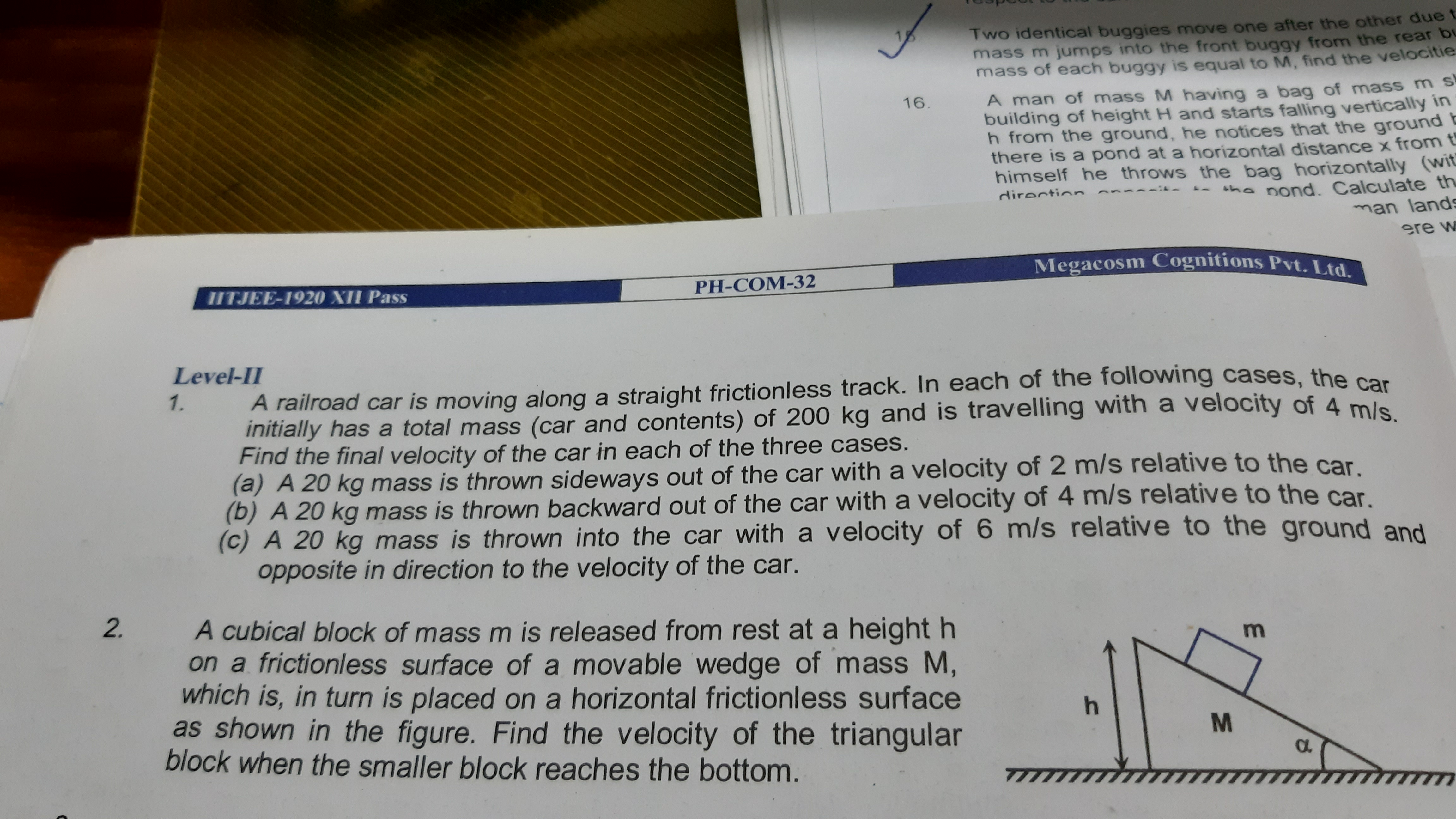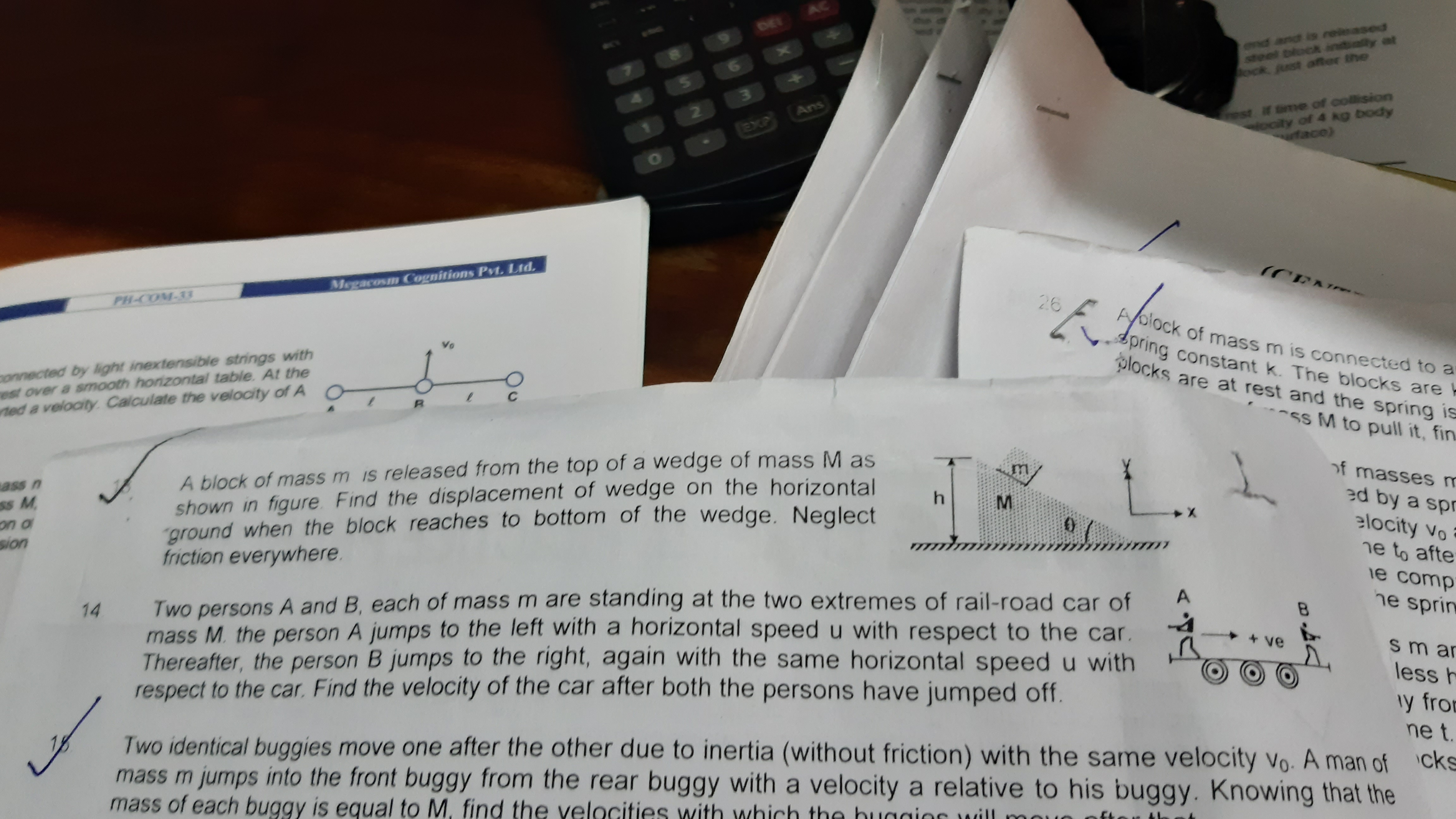Q14)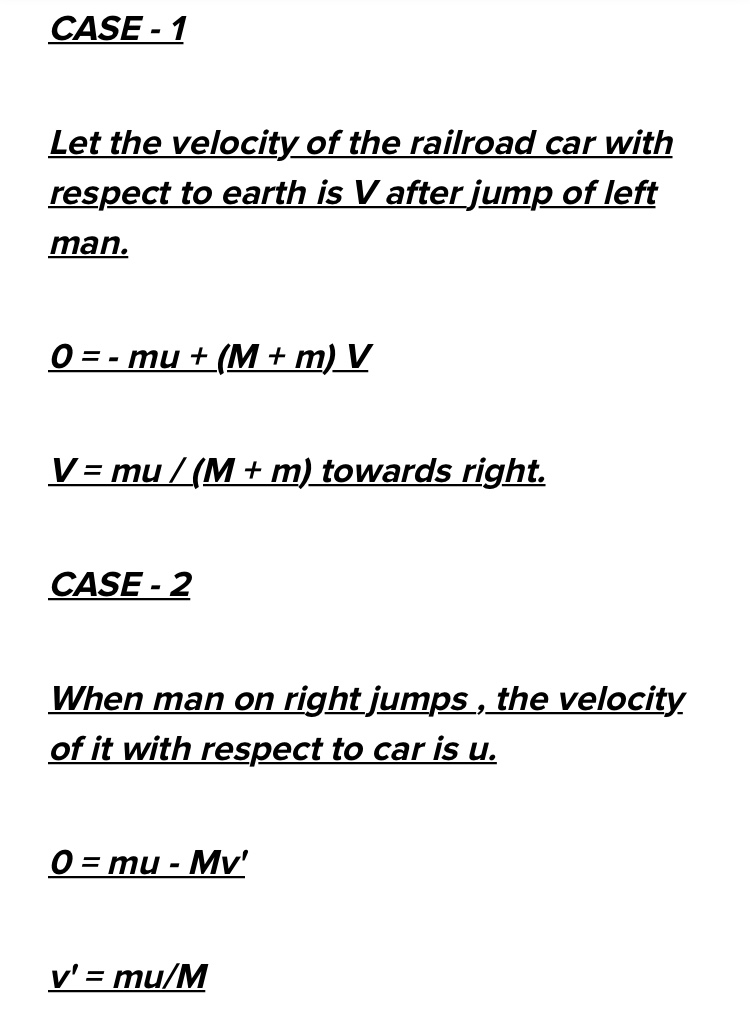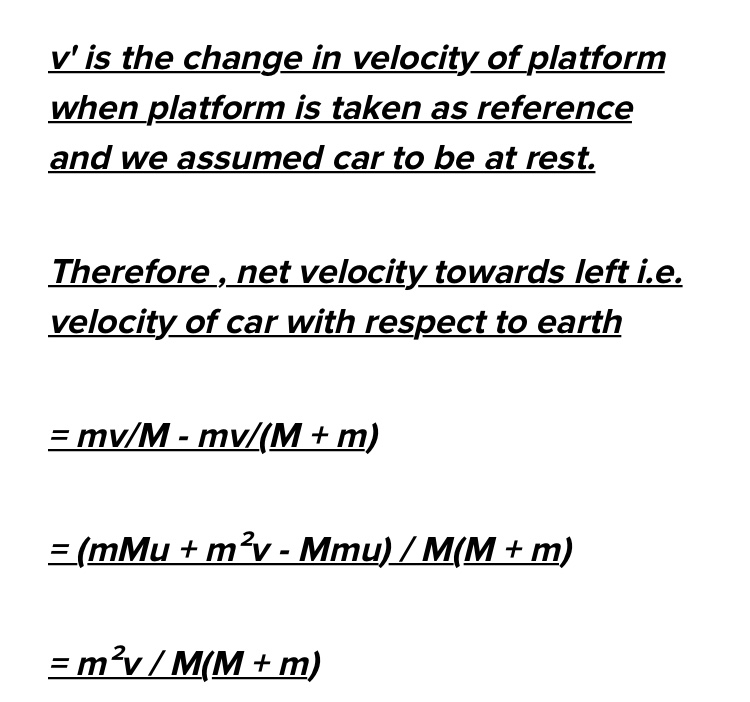Q2)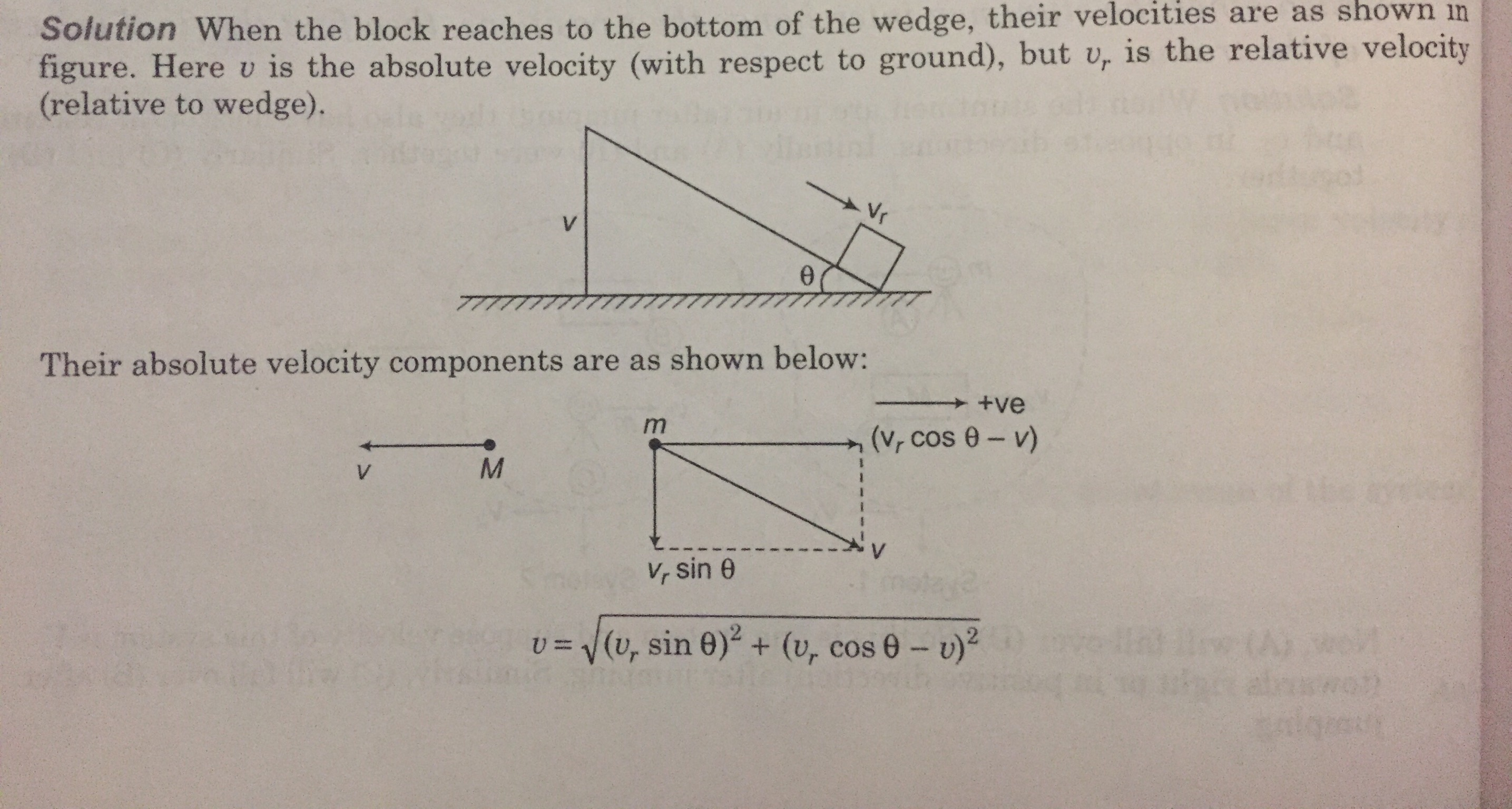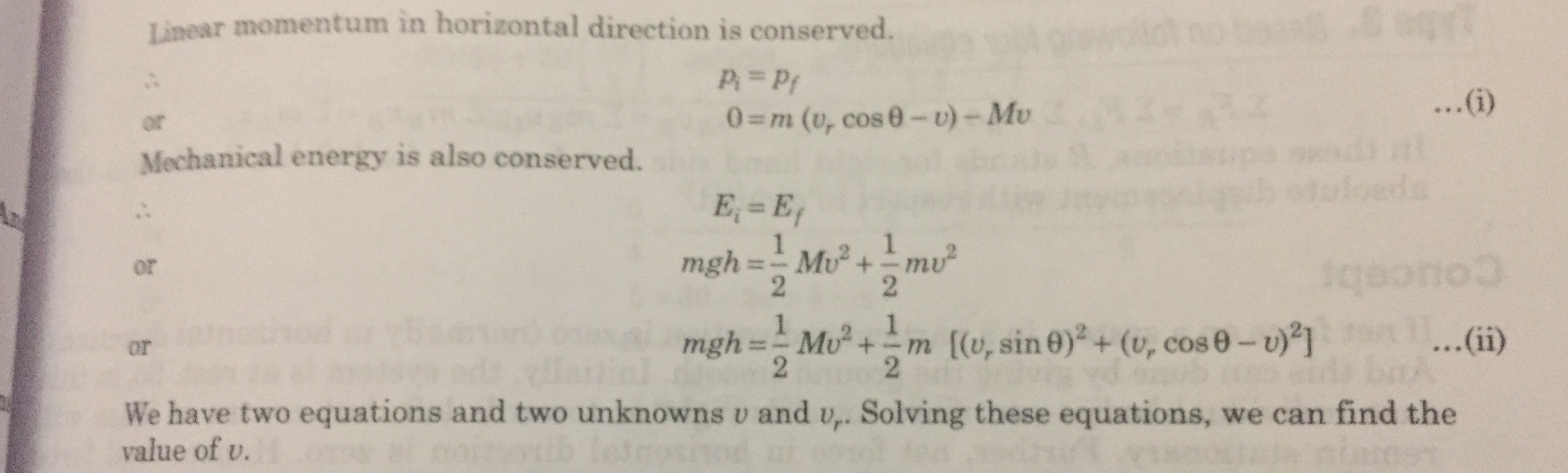Ok

There is some mistake in 14th answer as the answer given in answer key i am uploading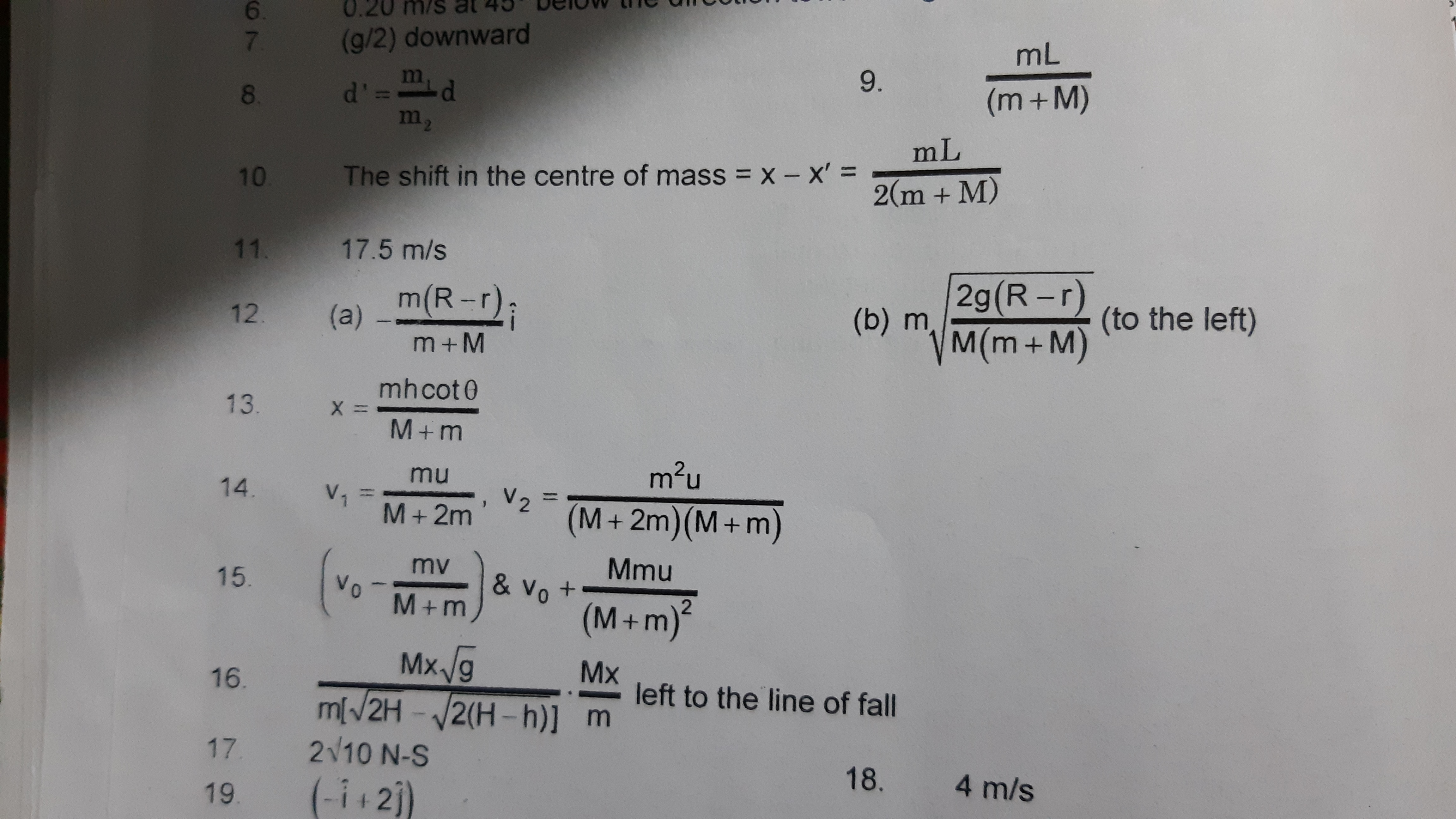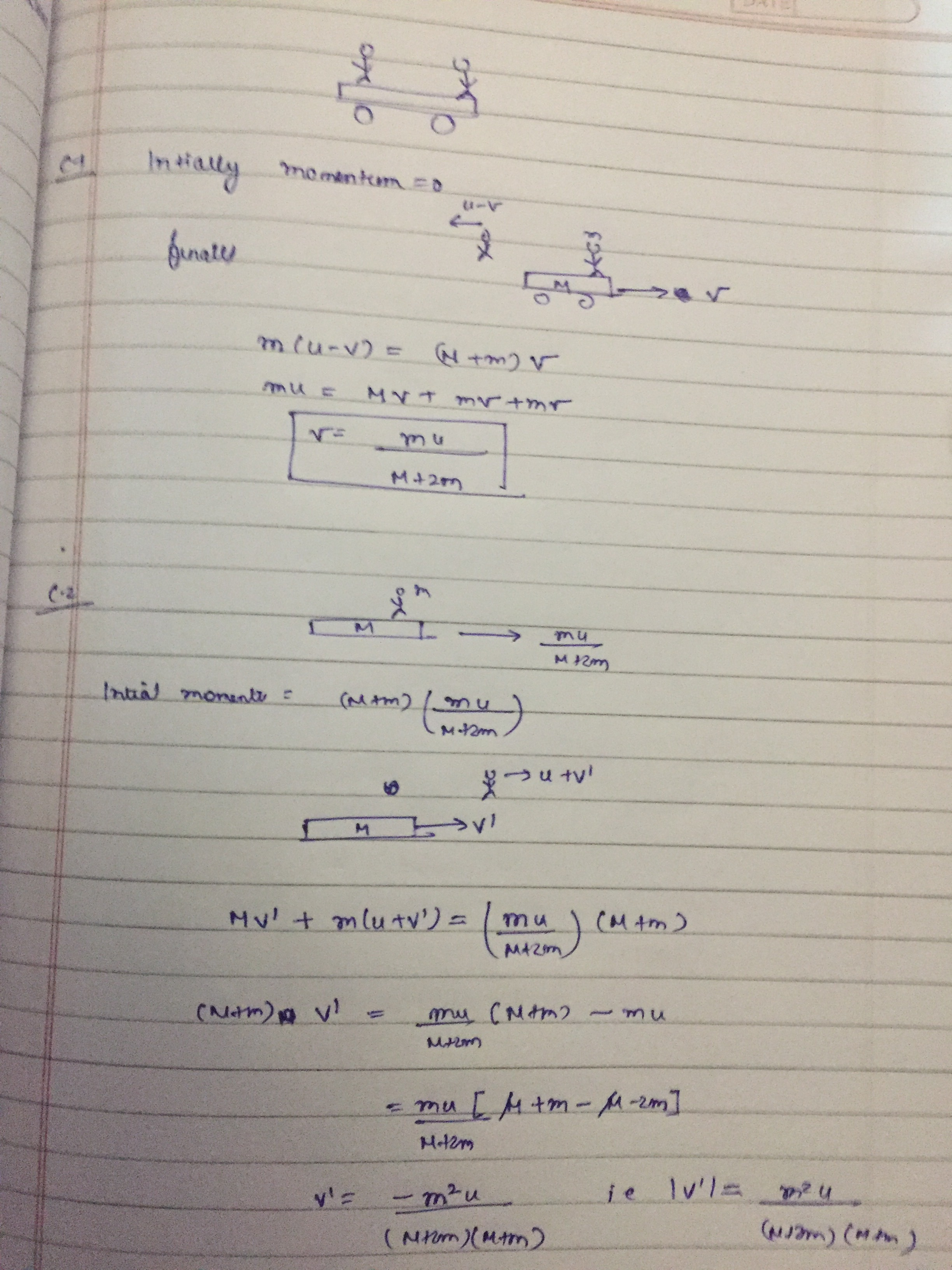Thanks

1 Like

This solution is wrong

What’s wrong in it ? Can you please elaborate

Concept is correct maybe but velocity cannot be calculated by this method i have done by another method

Velocity can surely be calculated by this methods.
What’s that different method that you used to solve this problem ? Did you get the correct answer ? Please show your workings

Yes i got correct answer as per in answer key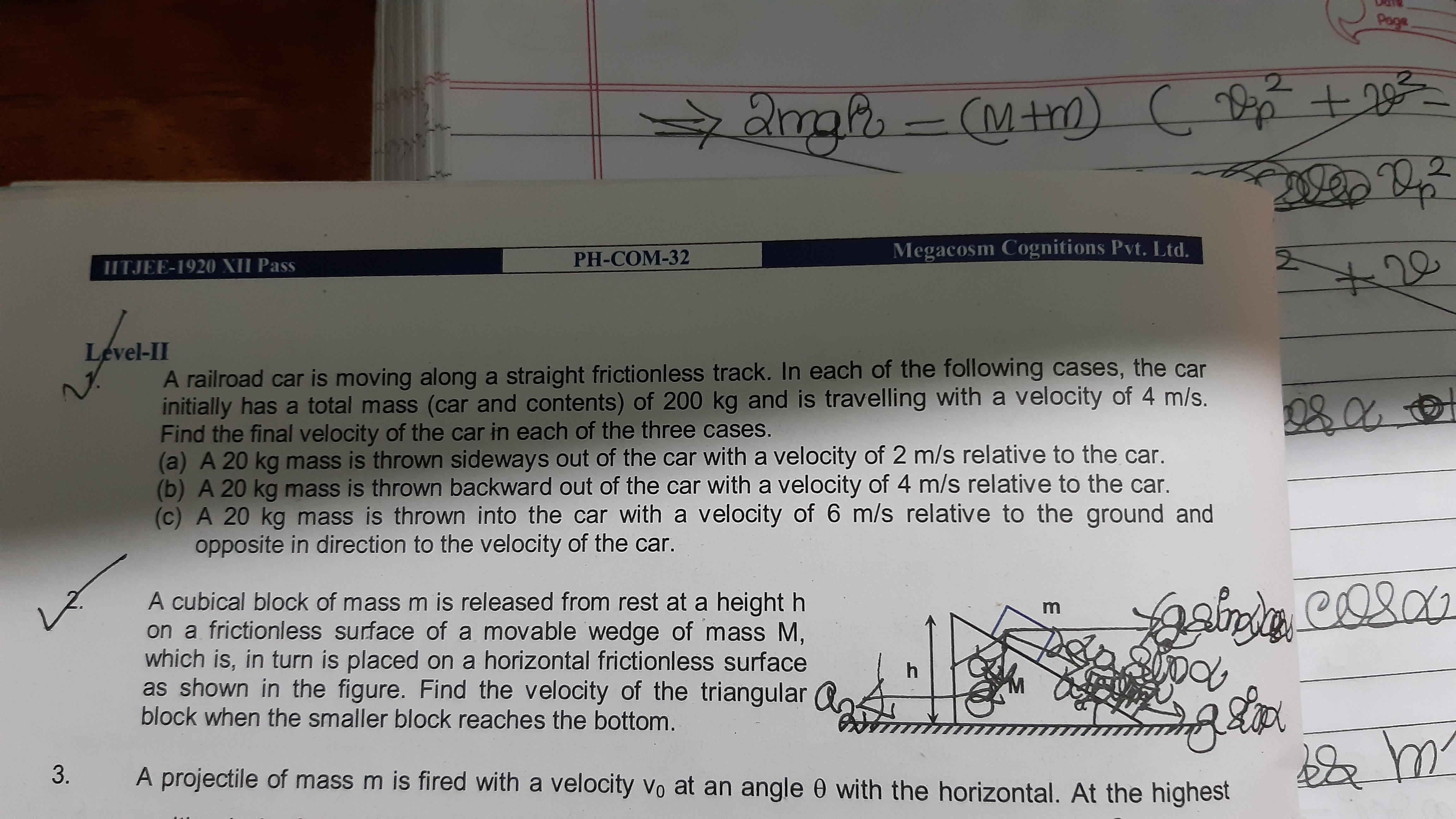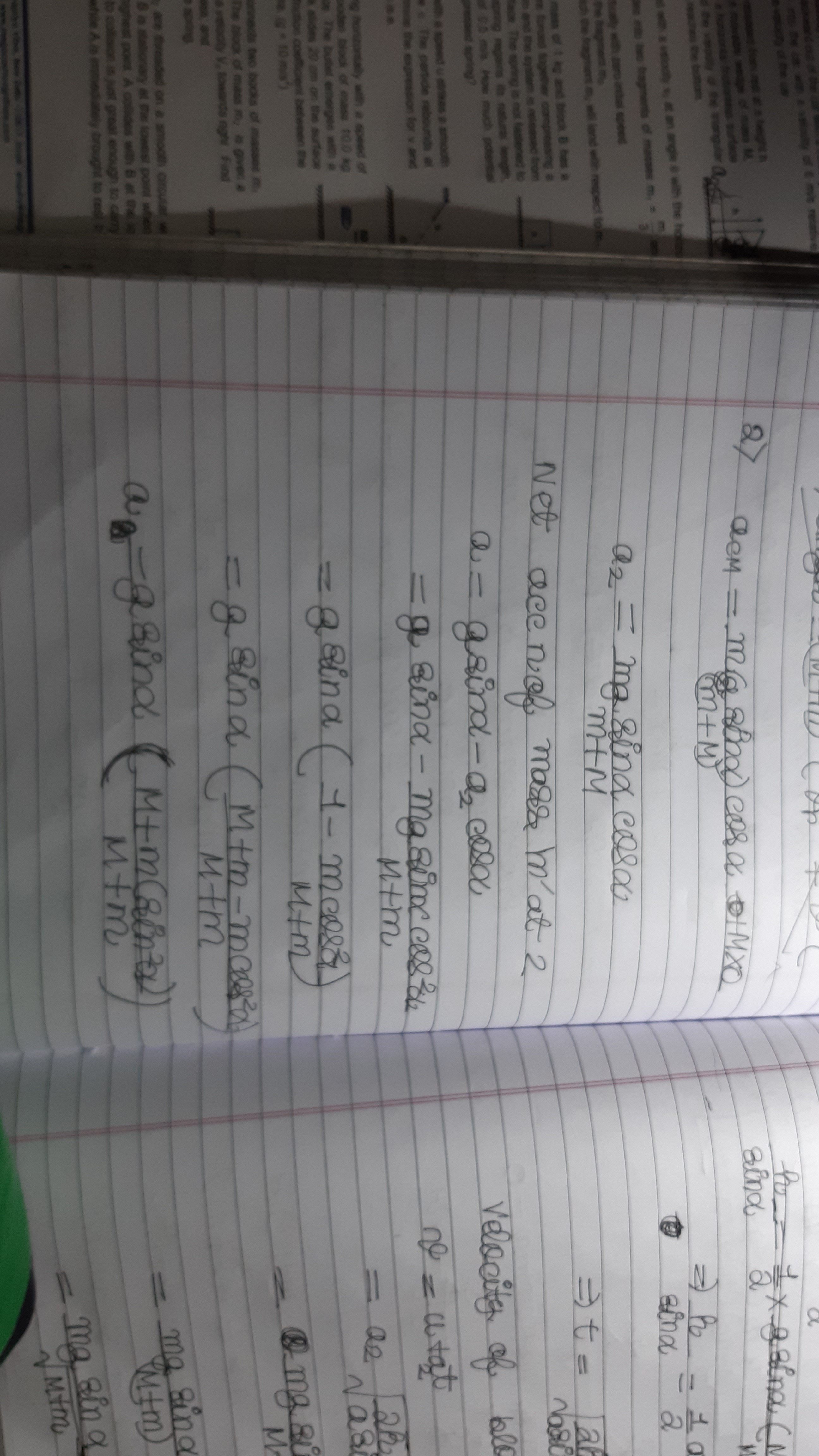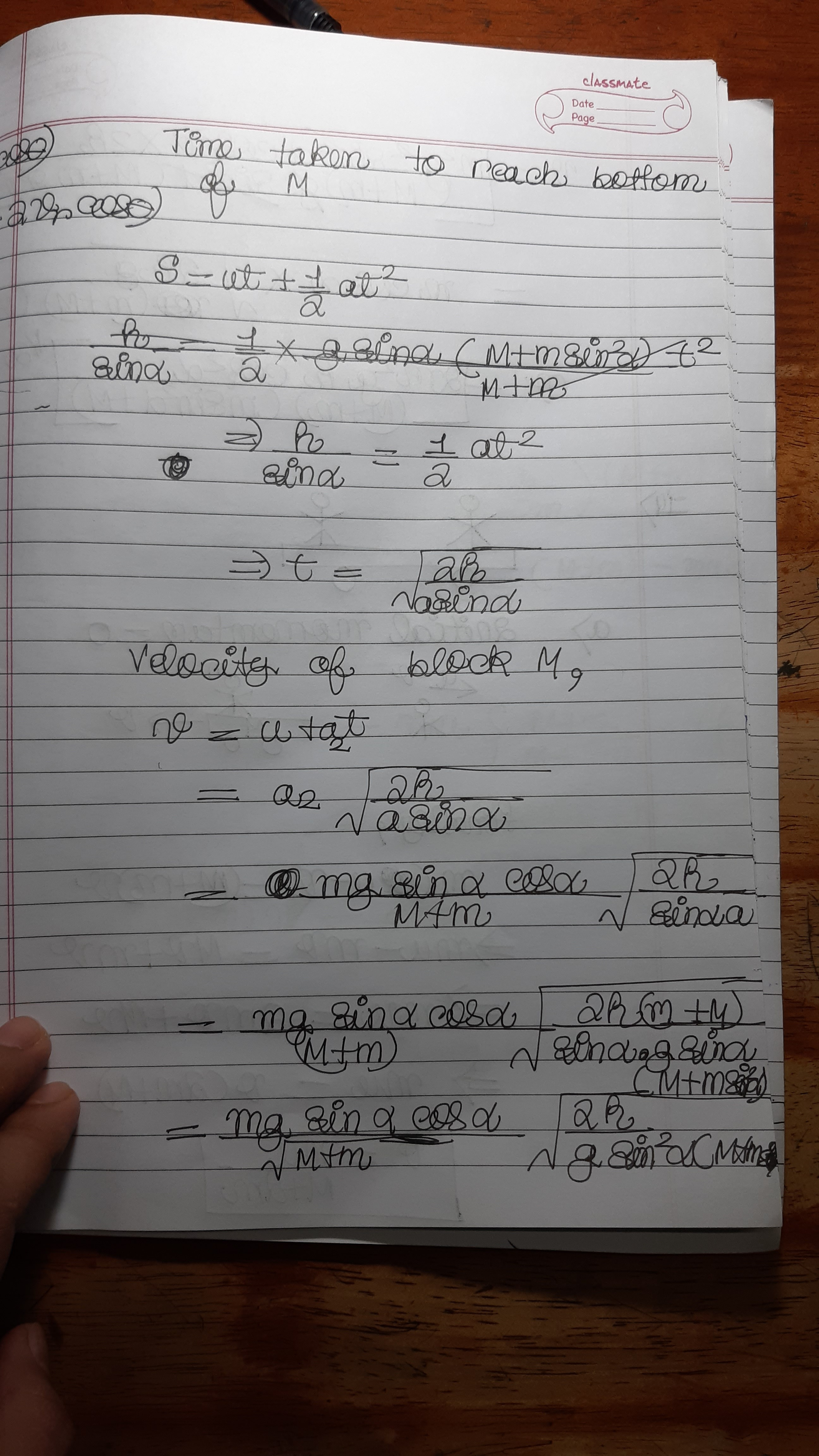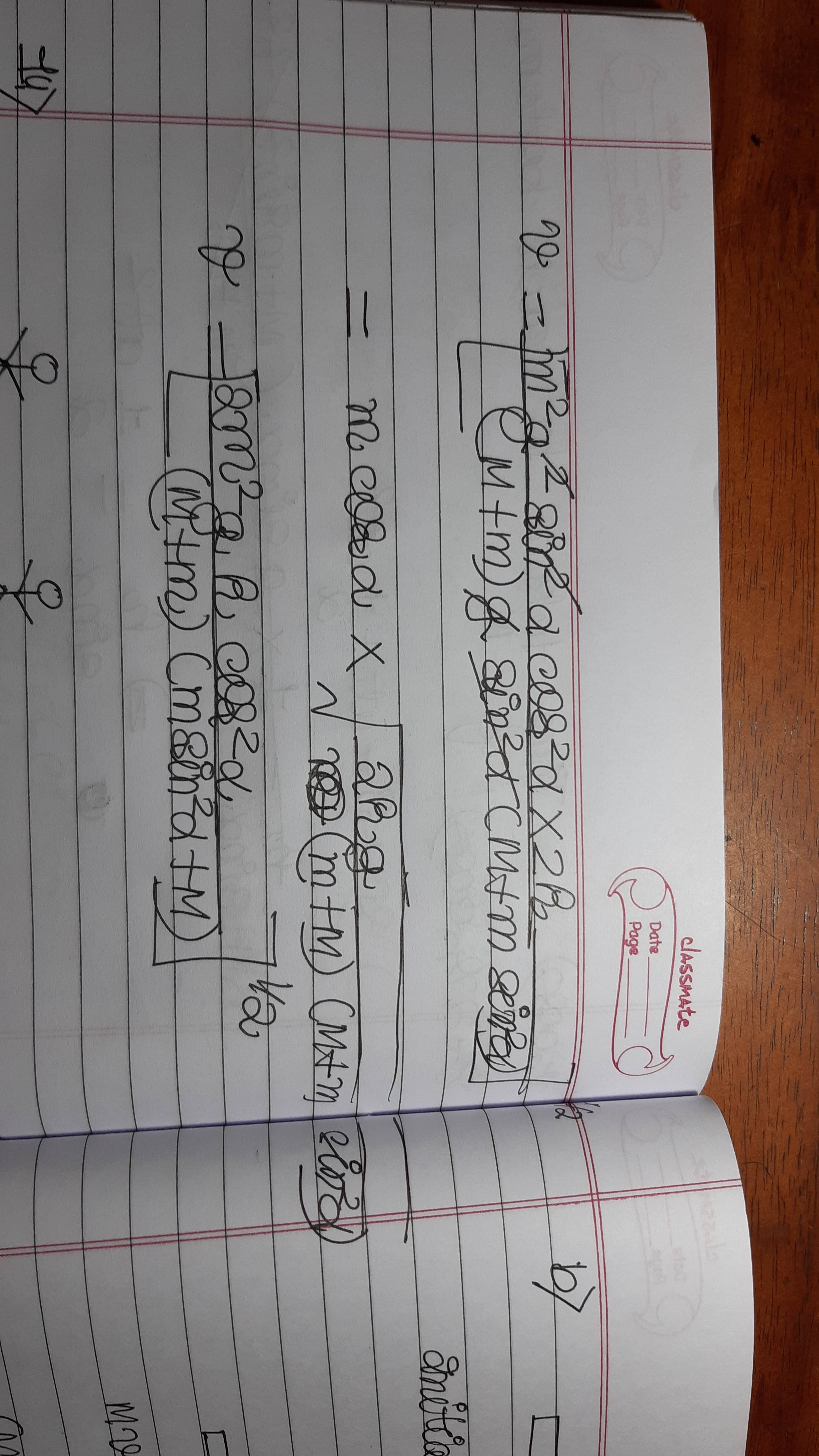@abhijit_2020 the method shown in post#4 is correct and you can also calculate the value of velocity from there

And coming to your solution, I don’t think it’s correct
Because the way you have calculated the acceleration of block of mass m seems completely wrong to me .
Also to get acceleration of smaller block along x you have taken cosѲ componet of gsinѲ and written it as gsinѲcosѲ which is wrong ,because there would also be sinѲ component of gcosѲ and hence net acceleration =0 in the x direction of block of mass m due to gravity will be zero
Simply you can understand it as mg is acting vertically downward so there can’t be acceleration due to some component of gravity in x direction

@Shwetanshu_2018 we cannot take components component....that is wrong

@Akash_2019 It’s nothing like we can’t take component’s component , we surely can , but here @abhijit_2020 has not taken the component properly that’s the thing

@Akash_2019
The method explained in the above post seems completely wrong !
What he has done is taken a component’s component in the y direction and claimed it to be Net force due to F in y direction that isn’t true .
For example let F=5i then it can also be written as 7i-2i what he has done is basically said we consider 7i not that is not equal to 5i
This doesn’t make any sense . If you want net force in the ‘i’ direction then you have to consider all the components of force f along ‘i’

Let me explain you the same example which was presented in the post you posted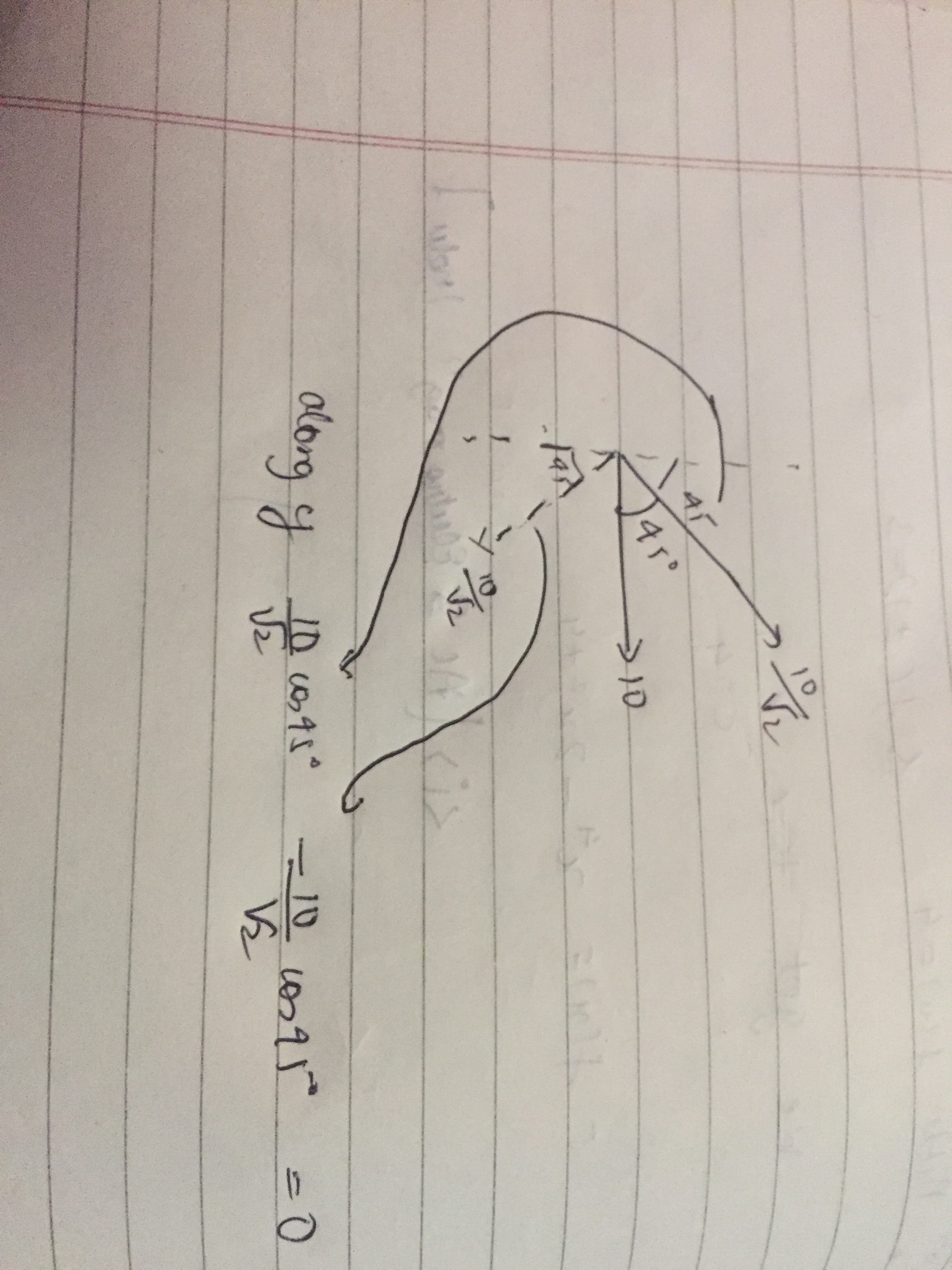To get actual net value of F in some direction , you’ll have to consider each component’s component in that direction

Question 2,5 and 10